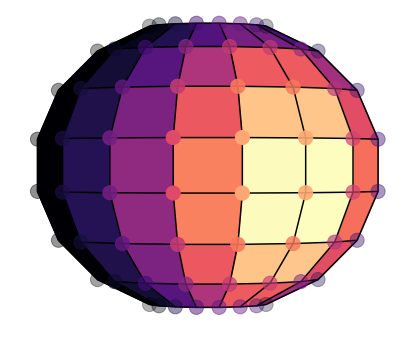# Alternative to plot_surface

plot_surface makes several polygons using the data points as vertices. Is it possible to instead plot similar polygons, but with the data points at the center?

For example, I would like the polygons of the plot below to be centered on where the circles are:code for the plot above:

fig = plt.figure(figsize=(7,6),facecolor=‘white’)
ax = plt.axes(projection=‘3d’)
norm = plt.Normalize(vmin=TOA_temp_arr[:,0].min(), vmax=TOA_temp_arr[:,0].max())
ax.plot_surface(x,y,z,facecolors=cmap(norm(TOA_temp_arr[50,0])),cstride=1,rstride=1,cmap=‘magma’)
ax.plot_wireframe(x,y,z,color=‘black’)
ax.scatter(x,y,z, c=colors_arr,cmap=‘magma’,s=200)

Not directly, but its not too hard to do a 2-D interpolation and get the values for the vertices from the values at the center. scipy.interpolate.interp2d — SciPy v1.7.0 Manual